The OpenFOAM FoundationRandom Class Reference

Random number generator. More...

## Public Member Functions

Random (const label s)
Construct from a seed. More...

~Random ()
Destructor. More...

scalar scalar01 ()
Advance the state and return a scalar sample from a uniform. More...

scalar scalarAB (const scalar a, const scalar b)
Advance the state and return a scalar sample from a uniform. More...

scalar scalarNormal ()
Advance the state and return a scalar sample from a normal. More...

template<class Type >
Type sample01 ()
Advance the state and return a sample of a given type from a. More...

template<class Type >
Type sampleAB (const Type &a, const Type &b)
Advance the state and return a sample of a given type from a. More...

template<class Type >
Type sampleNormal ()
Advance the state and return a sample of a given type from a. More...

scalar globalScalar01 ()
Advance the state and return a scalar sample from a uniform. More...

template<>
scalar sample01 ()

template<>
label sample01 ()

template<>
scalar sampleAB (const scalar &a, const scalar &b)

template<>
label sampleAB (const label &a, const label &b)

template<>
scalar sampleNormal ()

template<>
Foam::scalar sample01 ()

template<>
Foam::label sample01 ()

template<>
Foam::scalar sampleAB (const scalar &a, const scalar &b)

template<>
Foam::label sampleAB (const label &a, const label &b)

template<>
Foam::scalar sampleNormal ()

## Detailed Description

Random number generator.

This is a clone of the drand48 algorithm. This is significantly quicker than drand48, presumably due to the compiler inlining the sampling methods. It is also significantly quicker than the standard library linear congruential engine, as it does not use Schrage's algorithm to prevent overflow.

See http://pubs.opengroup.org/onlinepubs/007908775/xsh/drand48.html for details of the seeding and iteration sequence.

Source files

Definition at line 57 of file Random.H.

## ◆ Random()

 Random ( const label s )
inline

Construct from a seed.

Definition at line 41 of file RandomI.H.

## ◆ ~Random()

 ~Random ( )
inline

Destructor.

Definition at line 51 of file RandomI.H.

## ◆ scalar01()

 Foam::scalar scalar01 ( )
inline

Advance the state and return a scalar sample from a uniform.

distribution between zero and one

Definition at line 57 of file RandomI.H.

Here is the caller graph for this function: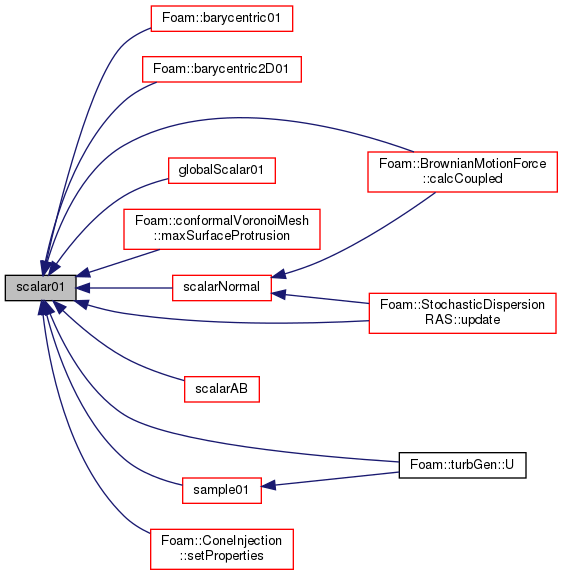## ◆ scalarAB()

 Foam::scalar scalarAB ( const scalar a, const scalar b )
inline

Advance the state and return a scalar sample from a uniform.

distribution between two limits

Definition at line 63 of file RandomI.H.

References Random::scalar01().

Referenced by uniform::sample(), Random::sampleAB(), and patchInjectionBase::setPositionAndCell().

Here is the call graph for this function: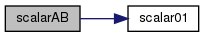Here is the caller graph for this function: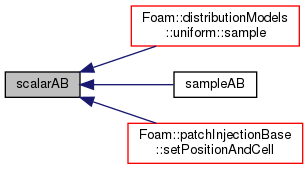## ◆ scalarNormal()

 Foam::scalar scalarNormal ( )

Advance the state and return a scalar sample from a normal.

distribution with mean zero and standard deviation one

Definition at line 31 of file Random.C.

References f(), Foam::log(), Random::scalar01(), Foam::sqr(), and Foam::sqrt().

Here is the call graph for this function: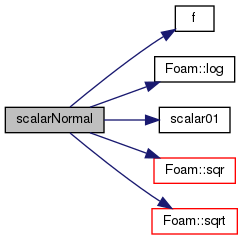Here is the caller graph for this function: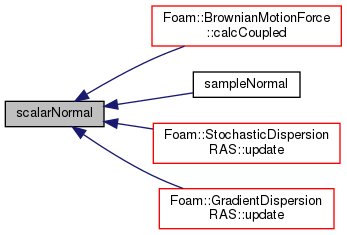## ◆ sample01() [1/5]

 Type sample01 ( )
inline

Advance the state and return a sample of a given type from a.

uniform distribution between zero and one

Definition at line 70 of file RandomI.H.

References Random::scalar01().

Here is the call graph for this function: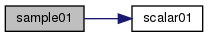Here is the caller graph for this function: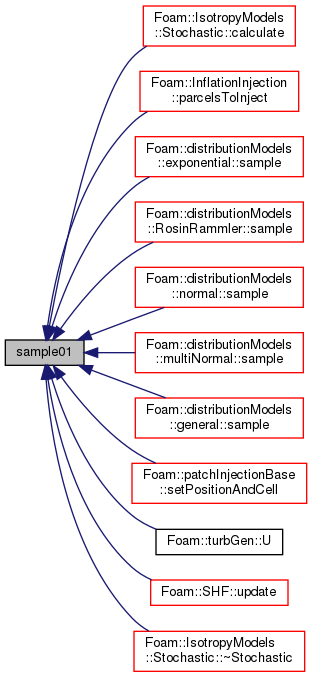## ◆ sampleAB() [1/5]

 Type sampleAB ( const Type & a, const Type & b )
inline

Advance the state and return a sample of a given type from a.

uniform distribution between two limits

Definition at line 98 of file RandomI.H.

References Foam::cmptMultiply().

Here is the call graph for this function: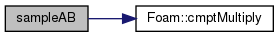Here is the caller graph for this function: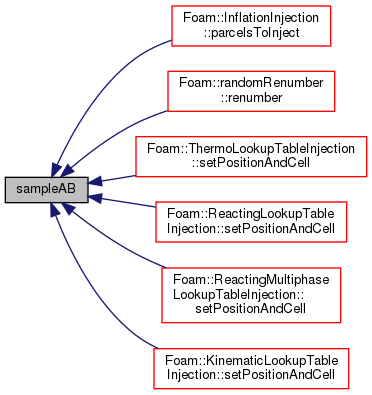## ◆ sampleNormal() [1/3]

 Type sampleNormal ( )
inline

Advance the state and return a sample of a given type from a.

normal distribution with mean zero and standard deviation one

Definition at line 119 of file RandomI.H.

References Random::scalarNormal().

Here is the call graph for this function: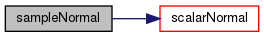## ◆ globalScalar01()

 Foam::scalar globalScalar01 ( )

Advance the state and return a scalar sample from a uniform.

distribution between zero and one. Synchronises across all cores. Use of this is discouraged. It is expensive and introduces non-randomness in all cores other then the master.

Definition at line 94 of file Random.C.

References UPstream::master(), Random::scalar01(), and Pstream::scatter().

Here is the call graph for this function: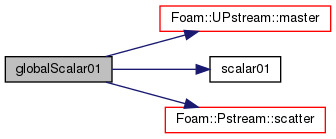Here is the caller graph for this function: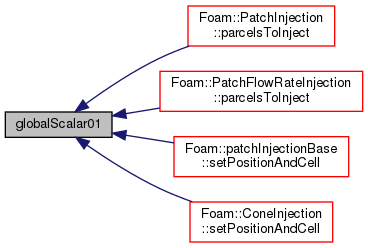## ◆ sample01() [2/5]

 scalar sample01 ( )
inline

## ◆ sample01() [3/5]

 label sample01 ( )
inline

## ◆ sampleAB() [2/5]

 scalar sampleAB ( const scalar & a, const scalar & b )
inline

## ◆ sampleAB() [3/5]

 label sampleAB ( const label & a, const label & b )
inline

## ◆ sampleNormal() [2/3]

 scalar sampleNormal ( )
inline

## ◆ sample01() [4/5]

 Foam::scalar sample01 ( )
inline

Definition at line 84 of file RandomI.H.

References Random::scalar01().

Here is the call graph for this function:## ◆ sample01() [5/5]

 Foam::label sample01 ( )
inline

Definition at line 91 of file RandomI.H.

## ◆ sampleAB() [4/5]

 Foam::scalar sampleAB ( const scalar & a, const scalar & b )
inline

Definition at line 105 of file RandomI.H.

References Random::scalarAB().

Here is the call graph for this function: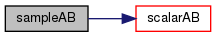## ◆ sampleAB() [5/5]

 Foam::label sampleAB ( const label & a, const label & b )
inline

Definition at line 112 of file RandomI.H.

## ◆ sampleNormal() [3/3]

 Foam::scalar sampleNormal ( )
inline

Definition at line 133 of file RandomI.H.

References Random::scalarNormal().

Here is the call graph for this function:The documentation for this class was generated from the following files: